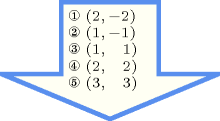## 5.1. abs_value

Origin

Arithmetic.

Constraint

$\mathrm{𝚊𝚋𝚜}_\mathrm{𝚟𝚊𝚕𝚞𝚎}\left(𝚈,𝚇\right)$

Usual name

$\mathrm{𝚊𝚋𝚜}$

Synonym

$\mathrm{𝚊𝚋𝚜𝚘𝚕𝚞𝚝𝚎}_\mathrm{𝚟𝚊𝚕𝚞𝚎}$.

Arguments
 $𝚈$ $\mathrm{𝚍𝚟𝚊𝚛}$ $𝚇$ $\mathrm{𝚍𝚟𝚊𝚛}$
Restriction
$𝚈\ge 0$
Purpose

Enforce the fact that the first variable is equal to the absolute value of the second variable.

Example
$\left(8,-8\right)$

The $\mathrm{𝚊𝚋𝚜}_\mathrm{𝚟𝚊𝚕𝚞𝚎}$ constraint holds since 8 is equal to $|-8|$.

All solutions

Figure 5.1.1 gives all solutions to the following non ground instance of the $\mathrm{𝚊𝚋𝚜}_\mathrm{𝚟𝚊𝚕𝚞𝚎}$ constraint: $𝚈\in \left[1,6\right],$ $𝚇\in \left[-2,3\right],$ $\mathrm{𝚊𝚋𝚜}_\mathrm{𝚟𝚊𝚕𝚞𝚎}$$\left(𝚈,𝚇\right)$.

##### Figure 5.1.1. All solutions corresponding to the non ground example of the $\mathrm{𝚊𝚋𝚜}_\mathrm{𝚟𝚊𝚕𝚞𝚎}$ constraint of the All solutions slotArg. properties

Functional dependency: $𝚈$ determined by $𝚇$.

Systems

abs in Choco, abs in Gecode.